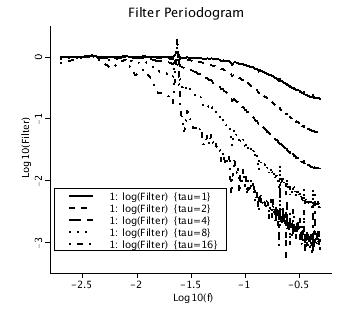# OperatorsAsFilters

Model number
0357

A series consisting of uniformly distributed random numbers between -1 and 1 is passed through a 1st order operator.

## Description

```A series is composed of uniformly distributed random numbers
between -1 and 1. It is used as the input to a 1st order
operator which acts as a low pass filter. The periodogram is
calculated for the input and output. The ratio (periodogram of
the output divided by periodogram of the input) is a low pass
filter. ```The filter response of a first order operator is displayed for tau = 1, 2, 4, 8, and 16. The data spacing is 1 unit.

## Equations

None.

The equations for this model may be viewed by running the JSim model applet and clicking on the Source tab at the bottom left of JSim's Run Time graphical user interface. The equations are written in JSim's Mathematical Modeling Language (MML). See the Introduction to MML and the MML Reference Manual. Additional documentation for MML can be found by using the search option at the Physiome home page.